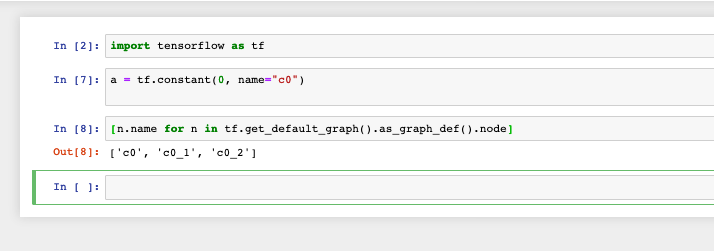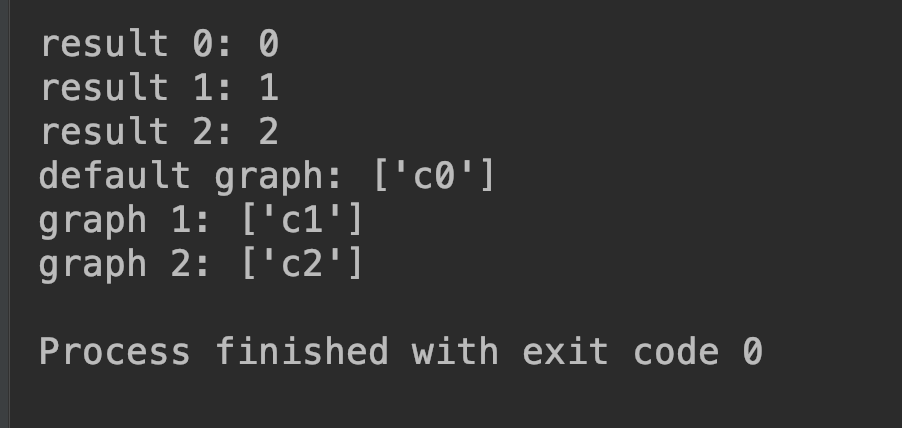#DAY 3
0

## 【03】新手常誤解的用法，tensorflow多個graph處理(如上圖，當你在 jupyter notebook 對第二個 cell 按了好幾次執行，其實也在 graph 裡產生很多個 c0常數)

Okay 那我們該如何管理這些graph呢？

tf.get_default_graph()

with tf.Graph().as_default()

``````tf.constant(0, name="c0")

with tf.Graph().as_default() as g1:
tf.constant(1, name="c1")

with tf.Graph().as_default() as g2:
tf.constant(2, name="c2")
``````

``````sess0 = tf.Session()
sess1 = tf.Session(graph=g1)
sess2 = tf.Session(graph=g2)

t0 = tf.get_default_graph().get_tensor_by_name('c0:0')
t1 = sess1.graph.get_tensor_by_name('c1:0')
t2 = sess2.graph.get_tensor_by_name('c2:0')

result0 = sess0.run(t0)
result1 = sess1.run(t1)
result2 = sess2.run(t2)

print("result 0: {}".format(result0))
print("result 1: {}".format(result1))
print("result 2: {}".format(result2))

print("default graph: {}".format([n.name for n in tf.get_default_graph().as_graph_def().node]))
print("graph 1: {}".format([n.name for n in g1.as_graph_def().node]))
print("graph 2: {}".format([n.name for n in g2.as_graph_def().node]))

sess0.close()
sess1.close()
sess2.close()
``````github原始碼